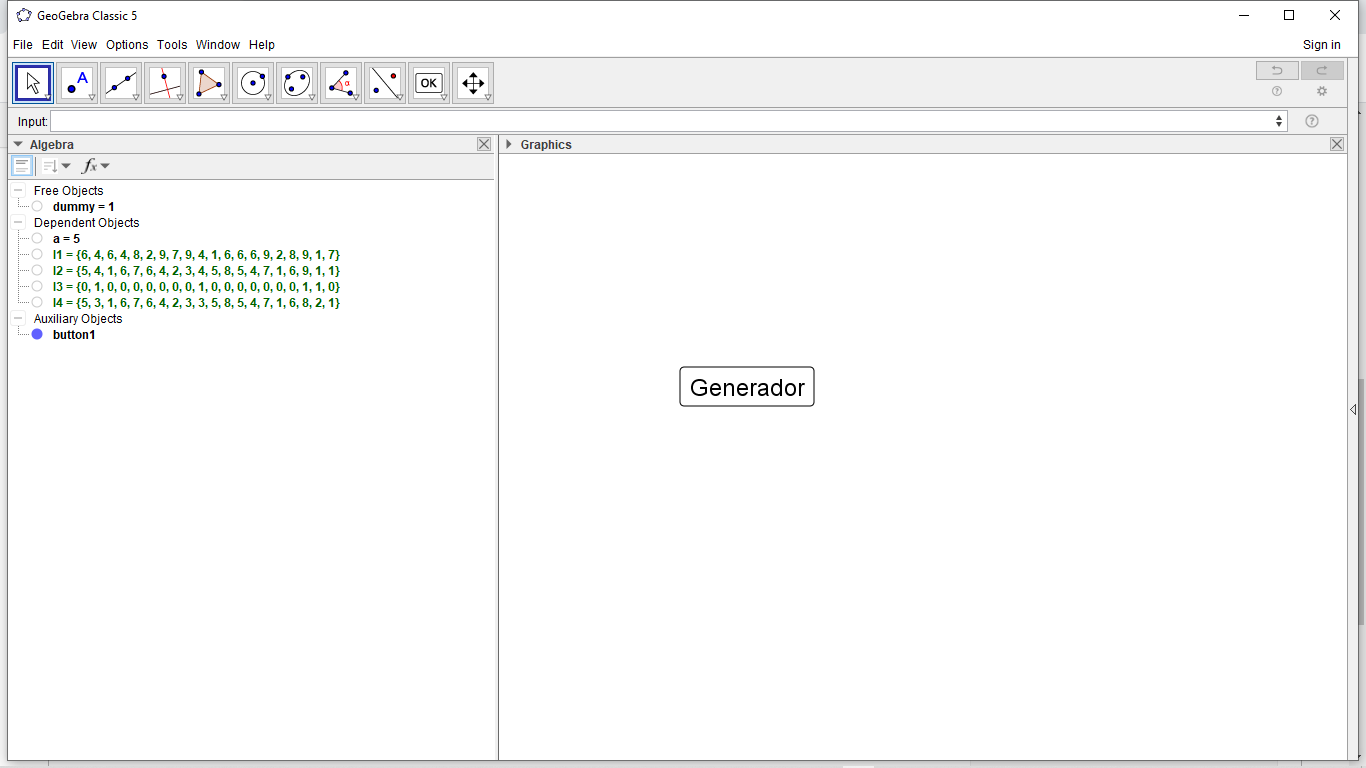# How to generate two lists with random elements without repeating the values of elements in the same

jospercomp shared this question 1 year ago

l1={1,7,3,8,2,6}

l2={3,8,4,6,3,4}now I understand

l1 = Sequence(13) - 6 \ {0} for numbers -5..7 but zero

l2 = Sequence(RandomElement(l1), k, 1, 20)

l3 = Sequence(RandomElement(l1 \ {l2(k)}), k, 1, 20)

l4 = Sequence(RandomElement(l1 \ {l2(k), l3(k)}), k, 1, 20) etc1

shuffle(sequence(n))

or

shuffle(list)1

l1=Sequence(dummy RandomBetween(1, 9), i, 1, 20)

l2=Sequence(dummy RandomBetween(1, 9), i, 1, 20)

l3=Sequence(If(Element(l1, i) ≟ Element(l2, i), 1, 0), i, 1, Length(l1))

l4=Sequence(If(Element(l3, i) ≟ 1, If(Max(l2) ≟ Element(l2, i), Element(l2, i) - 1, If(Min(l2) ≟ Element(l2, i), Element(l2, i) + 1, Element(l2, i) + (-1)^RandomBetween(1, 6))), Element(l2, i)), i, 1, Length(l1))12

Now I think I know what you actually want. Your original question was extremely vague. Based on your "solution", it looks a single list is allowed to have repeating values, and the second list is also allowed to have values form the first list but just not at the same position.

Your approach kind of does that but the elements in l4 are not uniformely distributed among the "allowed" values. Instead each element of l4 has a higher chance to be of distance 1 from the corresponding element in l1 than to be any other distance. Maybe that's not important for the kind of problem you need this for, but a modulo approach might be better here:

```l1 = Sequence(RandomBetween(1, 9), i, 1, 20)
l2 = Sequence(Mod(l1(i)+RandomBetween(0, 7), 9)+1, i, 1, 20)
```

or more generally (list of length n with random integers from a to b (whith b>a)):

```l1 = Sequence(RandomBetween(a, b), i, 1, n)
l2 = Sequence(a+Mod(l1(i)-a+RandomBetween(1, b-a), b-a+1), i, 1, n)
```

This way, for each element of the second list the values are uniformely distributed among all "allowed" values (i.e. among the values from a to b except the corresponding value in the first list).2

now I understand

l1 = Sequence(13) - 6 \ {0} for numbers -5..7 but zero

l2 = Sequence(RandomElement(l1), k, 1, 20)

l3 = Sequence(RandomElement(l1 \ {l2(k)}), k, 1, 20)

l4 = Sequence(RandomElement(l1 \ {l2(k), l3(k)}), k, 1, 20) etc